## Friday, December 2, 2011

### Why Direction Vectors Are Better Than Slopes

A direction vector is an ordered pair (in 2D) or triple (in 3D) which indicates direction by breaking it up into components that are perpendicular to each other.  A favorite trick of math people is to make the magnitude of this vector equal to 1, but it doesn’t always have to be – you can do a number of things with it even if its magnitude is random.

The first neat thing about direction vectors is that you don’t have to think about the special case of a vertical line.  When we use slopes, we are usually using either the point-slope form of a line or the slope-intercept form of a line.  These, respectively, are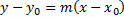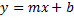where m is the slope of the line and b is the y-intercept.  In the world of slopes, you can’t deal with this without taking a special case because anything divided by zero is undefined.  For this application, maybe we could denote that as infinity, but infinity does not cooperate with ordinary numbers without a good deal of coaxing.  The workaround is to say x = c, where c is a constant.  More generally, we could use the general form of the equation: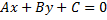If we had our rise and run for our slope as a and b, respectively, then m = a/b.  But if we put this into the point-slope form we get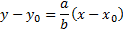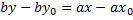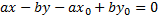If we do it this way, and b = 0, we can skip the whole division by zero thing.  Unless you blinked, you just learned what direction vectors are.  Okay, you probably blinked, so let me put that differently.

The values a and b are the components of our direction vector which can be expressed as (b, a).  The most fun thing we can do with a direction vector is rotate it.  For example, to rotate this vector counter-clockwise 90°, we would use (–a, b).  To rotate clockwise 90°, we would use (a, –b).  Both of these are, by definition, normal to our given direction vector.  You can see from the above equation that the general form equation of a line contains the normal vector.  To rotate a direction vector (b, a) counter-clockwise by θ, we take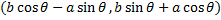This leads us to a useful way to treat direction vectors as complex numbers.  The above product is produced in complex numbers according to

The above rotation scheme(s) preserve the magnitude of the vector.  The complex multiplication representation can be convenient as a matter of notation and for programming in systems that support complex numbers – or so I believe.

Another really cool thing you can do with direction vectors is use parametric equations.  The parametric representation of a line (in 2D and 3D) offers some interesting benefits.